IF YOU CAN SEE THIS go to /sfa/Nuprl/Shared/Xindentation_hack_doc.html

### Sequents with Restricted Declarations - continued from Sequents with Type Conclusions

We continue elaborating on sequents having types as conclusions. A declaration may be annotated as the second one is in the following sequent:

1. x :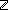2. y :[not for witness]
3. x+1<y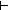{z:x<z & z<y }

The sequent above claims that there is some "witness" expression ???, not containing y free, such that

1. x :2. y :3. x+1<y???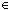{z:x<z & z<y }

in which case the witness y-1{z:x<z & z<y } would not suffice; however, the witness expression "x+1" would suffice since it does not use y.

Now, why do we care about restricting the use of declared variables from witnesses?
Recalling that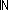k (i.e. {i:| 0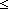i < k }) is our standard finite set of size k, consider the difference between the following two types of functions.

Thm* k: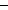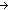x:(k List){y:(k+1)| y greater-bounds x }
Thm*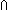k:x:(k List){y:(k+1)| y greater-bounds x }

where Def y greater-bounds x ==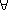i:||x||. x[i]<y,
and ||x|| denotes the length of a list,
and x[i] denotes the member at position i||x|| of list x,
the head of a list being considered position 0.

The first is the type of functions which, applied to two arguments, a number kand a list of numbers less than k, find a number no bigger than k, but bigger than every member of the list. It is of course quite trivial to find such a function-- just use k, as suggested in the proof.
The second, an Intersection Type, is the type of functions of one argument, a list of any type (k List) for any k, that return such an upper bound, but do not take k itself as an argument. Such functions must examine the list to come up with a bound.

The proofs given for the above two theorems begin similarly, producing the following subgoals respectively:

1. k :2. x :k List{y:(k+1)| y greater-bounds x }

1. k :[not for witness]
2. x :k List{y:(k+1)| y greater-bounds x }

the difference being simply that the upper sequent can be satisfied by witness "k", but the second requires a witness not involving "k", and indeed the proof can be seen to proceed by induction on list structure. Incidentally, note that the inference to 1. x : A ListB(x) fromB(nil) and 1. u : A  2. v : A List  3. B(v)B((u.v)) is justified by constructing the witness

Case of x; nil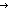p ; u.v, rec:rq(uvr)

from witnesses "p" and "q(uvr)" for the two premise sequents.

Representing Propositions as Types affords Further Uses of Restricted Declarations.

(March 2001 - sfa )